# Hard looking yet beautiful integral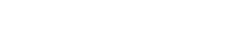Never judge a book by its cover: this is the case. This integral requires a bit of work and knowledge, as you need to know about the Gaussian integral (I covered it in these two posts: https://addjustabitofpi.com/gaussian-integral-using-feynmans-technique/https://addjustabitofpi.com/integral-of-e-xt-using-feynmans-technique/) and the most basic method of integration, u-substitution (https://addjustabitofpi.com/2019/10/05/integration-techniques-u-substitution/ & https://addjustabitofpi.com/2019/10/13/integration-techniques-u-substitution-part-2/). So let’s start!

Look at the Gaussian integral: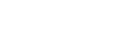Instead of having –x², we have -(a-x)²/4kt. If you think about it though, we can write this as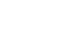so that, when substituting, we will get the form we know. So,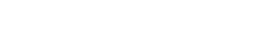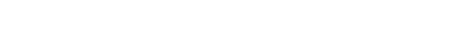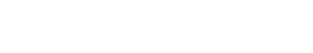As you can see, we can do some simplifications: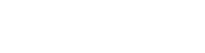but there is still something to do: since we substituted, we are now integrating with respect to u, therefore all x‘s must be written in terms of u; also, the bounds change, since those are for x.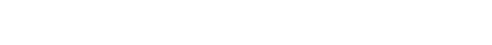I stopped at -x because we have e^-x inside the integral, therefore there’s no point in finding x and then inverting the sign again.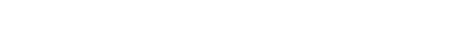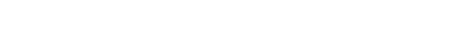Let’s put everything together: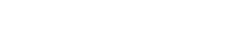Since we have the – sign in front of the integral, we can swap the bounds: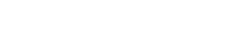There is still one thing we can do. e is raised to a sum, so we can write it as a product: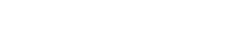e^-a is a constant (with respect to u), meaning we can move it outside the integral:Here instead, we can do the opposite:We have u², we have u… it looks like the expansion of the square of a binomial! To make things easier, let’s factor out the – sign: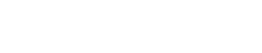Now we are going to “complete the square”: say you have x²-2x; you can see that the missing term in order to make a square is 4, so you can write it as x²-2x+4-4= (x-2)²-4. (-4 because if you simply add 4, the two things won’t be equivalent anymore). This is exactly what we are doing here. First though, let’s take that 4 out of the square root:The missing term is kt, so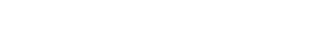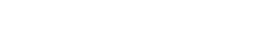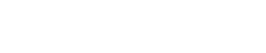At this point, we can perform another substitution: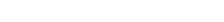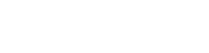This time the bounds stay the same, since nothing changes when you plug infinity and -infinity into u-sqrt(kt).

This is the Gaussian integral! And we saw here that this is equal to sqrt(pi).

Therefore,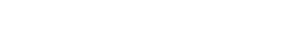Overall,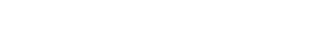And that’s it!

Hope you liked this post! If so, give it a like and leave a comment if you have any doubt, question, suggestions!

Join 31 other subscribers.